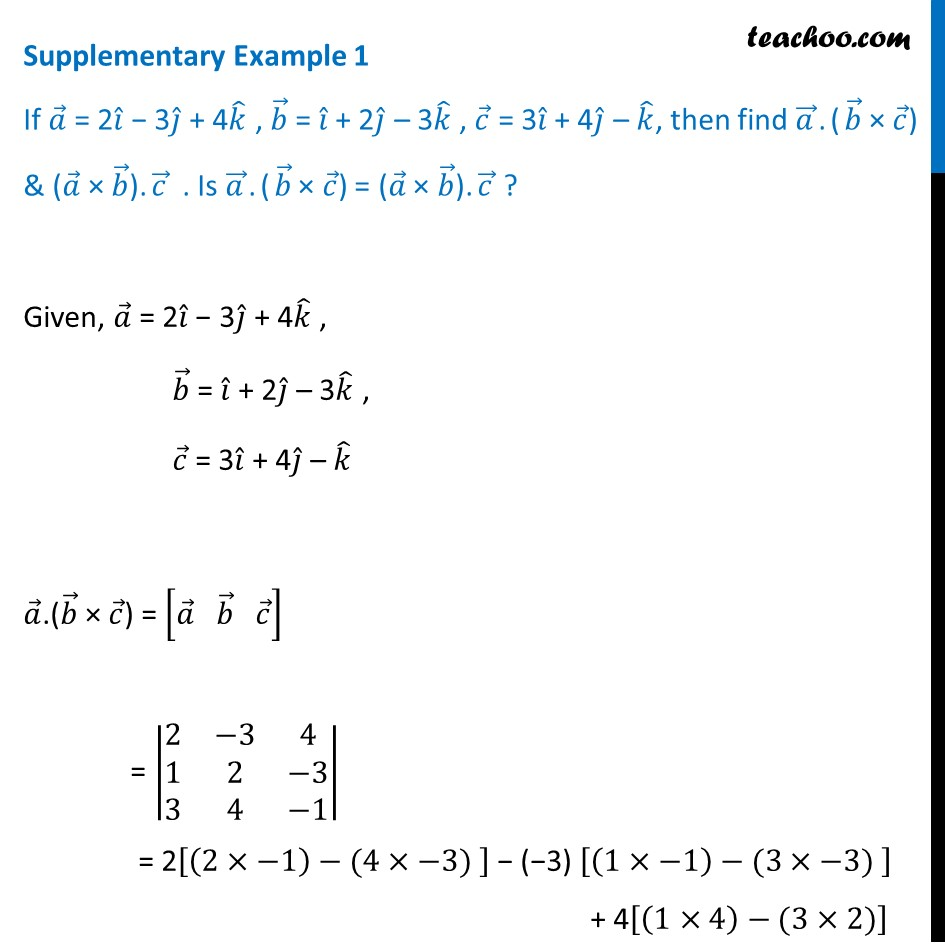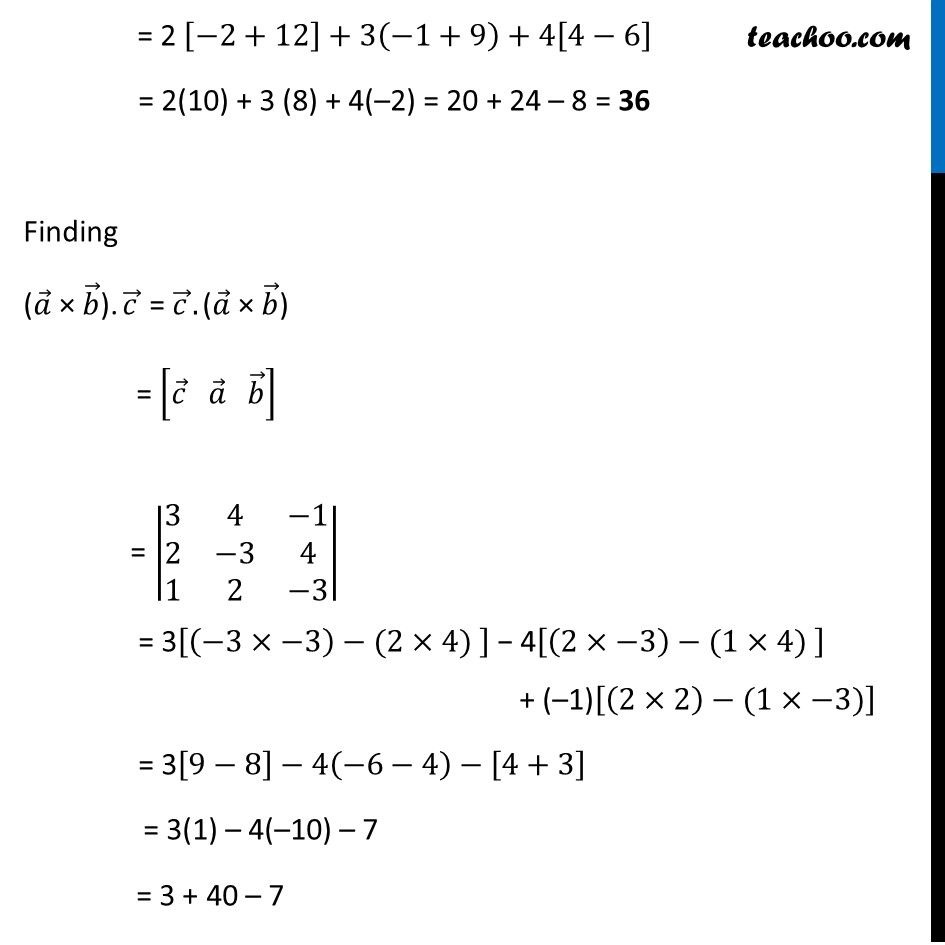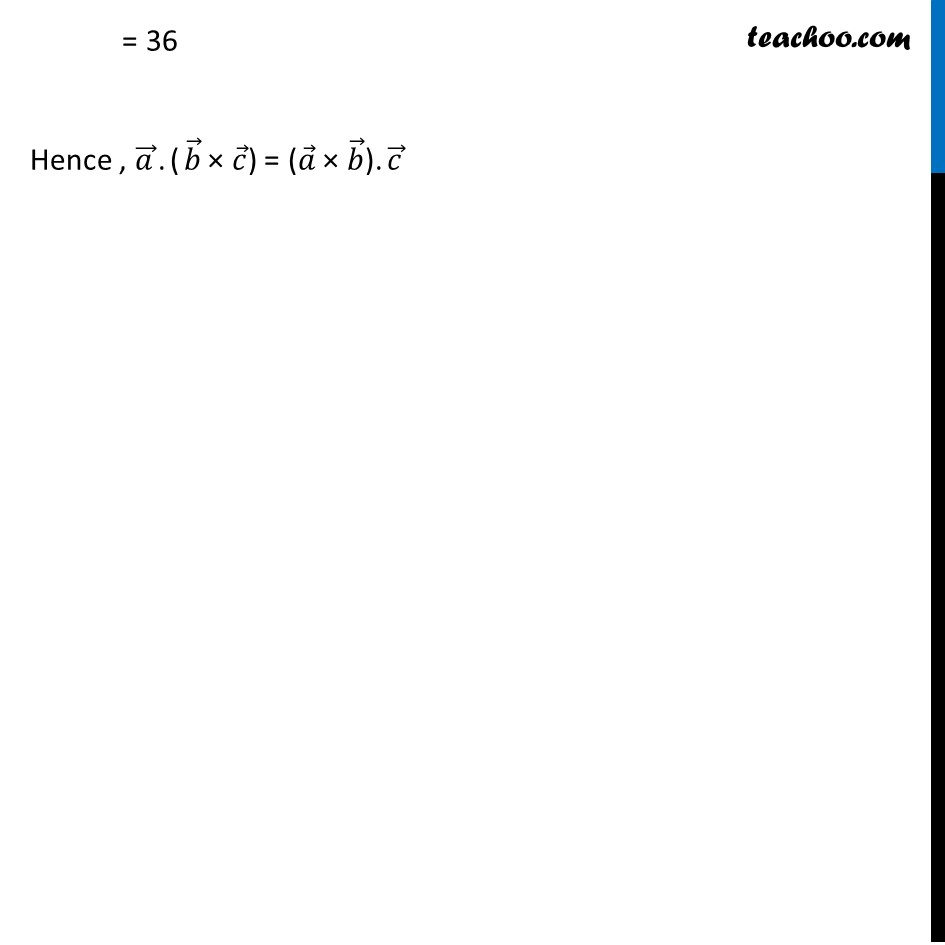Supplementary examples and questions from CBSE

Chapter 10 Class 12 Vector Algebra
Serial order wiseLearn in your speed, with individual attention - Teachoo Maths 1-on-1 Class

### Transcript

Supplementary Example 1 If 𝑎 ⃗ = 2𝑖 ̂ − 3𝑗 ̂ + 4𝑘 ̂ , 𝑏 ⃗ = 𝑖 ̂ + 2𝑗 ̂ – 3𝑘 ̂ , 𝑐 ⃗ = 3𝑖 ̂ + 4𝑗 ̂ – 𝑘 ̂, then find 𝑎 ⃗.(𝑏 ⃗ × 𝑐 ⃗) & (𝑎 ⃗ × 𝑏 ⃗).𝑐 ⃗ . Is 𝑎 ⃗.(𝑏 ⃗ × 𝑐 ⃗) = (𝑎 ⃗ × 𝑏 ⃗).𝑐 ⃗ ? Given, 𝑎 ⃗ = 2𝑖 ̂ − 3𝑗 ̂ + 4𝑘 ̂ , 𝑏 ⃗ = 𝑖 ̂ + 2𝑗 ̂ – 3𝑘 ̂ , 𝑐 ⃗ = 3𝑖 ̂ + 4𝑗 ̂ – 𝑘 ̂ 𝑎 ⃗.(𝑏 ⃗ × 𝑐 ⃗) = [𝑎 ⃗" " 𝑏 ⃗" " 𝑐 ⃗ ] = |■8(2&−3&[email protected]&2&−[email protected]&4&−1)| = 2[(2×−1)−(4×−3) ] − (−3) [(1×−1)−(3×−3) ] + 4[(1×4)−(3×2)] = 2 [−2+12]+3(−1+9)+4[4−6] = 2(10) + 3 (8) + 4(–2) = 20 + 24 – 8 = 36 Finding (𝑎 ⃗ × 𝑏 ⃗).𝑐 ⃗ = 𝑐 ⃗.(𝑎 ⃗ × 𝑏 ⃗) = [𝑐 ⃗" " 𝑎 ⃗" " 𝑏 ⃗ ] = |■8(3&4&−[email protected]&−3&[email protected]&2&−3)| = 3[(−3×−3)−(2×4) ] − 4[(2×−3)−(1×4) ] + (–1)[(2×2)−(1×−3)] = 3[9−8]−4(−6−4)−[4+3] = 3(1) – 4(–10) – 7 = 3 + 40 – 7 = 36 Hence , 𝑎 ⃗.(𝑏 ⃗ × 𝑐 ⃗) = (𝑎 ⃗ × 𝑏 ⃗).𝑐 ⃗